International
Tables for
Crystallography
Volume H
Powder diffraction
Edited by C. J. Gilmore, J. A. Kaduk and H. Schenk

International Tables for Crystallography (2018). Vol. H, ch. 3.8, pp. 325-327

## Section 3.8.2. Comparing 1D diffraction patterns

C. J. Gilmore,a G. Barra and W. Donga*

aDepartment of Chemistry, University of Glasgow, University Avenue, Glasgow, G12 8QQ, UK
Correspondence e-mail:  chris@chem.gla.ac.uk

### 3.8.2. Comparing 1D diffraction patterns

| top | pdf |

Comparing 1D diffraction patterns or spectra cannot be done by simply using the peaks and their relative intensities for a number of reasons:

 (1) The accurate determinations of the peak positions may be difficult, especially in cases where peak overlap occurs or there is significant peak asymmetry. (2) The hardware and the way in which the sample is prepared can also affect the d-spacing (or 2θ value) that is recorded for the peak. Shoulders to main peaks and broad peaks can also be problematic. (3) There is a subjective element to deciding how many peaks there are in the pattern, especially for weak peaks and noisy data. (4) Weak peaks may be discarded. This can affect the quantitative analysis of mixtures if one component diffracts weakly or is present only in small amounts. (5) Differences in sample preparation and instrumentation can lead to significant differences in the powder-diffraction patterns of near-identical samples. (6) Preferred orientation may be present: this is a very difficult and common problem. (7) The reduction of the pattern to point functions can also make it difficult to design effective algorithms.

In order to use the information contained within the full profile, algorithms are required that utilize each measured data point in the analysis. We use two correlation coefficients for the purpose of comparing PXRD patterns: the Pearson and the Spearman coefficients.

#### 3.8.2.1. Spearman's rank order coefficient

| top | pdf |

Consider two diffraction patterns, i and j, each with n measured points n((x1, y1), …, (xn, yn)). These are transformed to ranks R(xk) and R(yk). The Spearman test (Spearman, 1904) then gives a correlation coefficient (Press et al., 2007),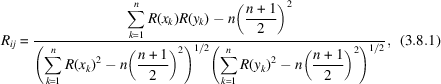where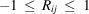.

#### 3.8.2.2. Pearson's r coefficient

| top | pdf |

Pearson's r is a parametric linear correlation coefficient widely used in crystallography. It has a similar form to Spearman's test, except that the data values themselves, and not their ranks, are used: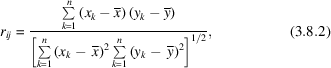where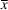and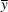are the means of intensities taken over the full diffraction pattern. Again, r can lie between −1.0 and +1.0.

Fig. 3.8.1shows the use of the Pearson and Spearman correlation coefficients (Barr et al., 2004a). In Fig. 3.8.1(a) r = 0.93 and R = 0.68. The high parametric coefficient arises from the perfect match of the two biggest peaks, but the much lower Spearman coefficient acts as a warning that there are unmatched regions in the two patterns. In Fig. 3.8.1(b) the situation is reversed: r = 0.79, whereas R = 0.90, and it can be seen that there is a strong measure of association with the two patterns, although there are some discrepancies in the region 15–35°. In Fig. 3.8.1(c) r = 0.66 and R = 0.22; in this case the Spearman test is again warning of missing match regions. Thus, the use of the two coefficients acts as a valuable balance of their respective properties when processing complete patterns. The Spearman coefficient is also robust in the statistical sense and useful in the case of preferred orientation.Figure 3.8.1 | top | pdf |The use of the Pearson (r) and Spearman (R) correlation coefficients to quantitatively match powder patterns: (a) r = 0.93, R = 0.68; (b) r = 0.79, R = 0.90; (c) r = 0.66, R = 0.22.

#### 3.8.2.3. Combining the correlation coefficients

| top | pdf |

Correlation coefficients are not additive, so it is invalid to average them directly; they need to be transformed into the Fisher Z value to give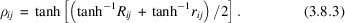#### 3.8.2.4. Full-profile qualitative pattern matching

| top | pdf |

Before performing pattern matching, some data pre-processing may be necessary. In order not to produce artefacts, this should be minimized. Typical pre-processing activities are:

 (1) The data are normalized such that the maximum peak intensity is 1.0. (2) The patterns need to be interpolated if necessary to have common increments in 2θ. High-order polynomials using Neville's algorithm can be used for this (Press et al., 2007). (3) If backgrounds are large they should be removed. High-throughput data are often very noisy because of low counting times and the sample itself. If this is the case, smoothing of the data can be carried out. The SURE (Stein's Unbiased Risk Estimate) thresholding procedure (Donoho & Johnstone, 1995; Ogden, 1997) employing wavelets is ideal for this task since it does not introduce potentially damaging artefacts, for example ringing around peaks (Barr et al., 2004a; Smrčok et al., 1999).

After pre-processing, which needs to be carried out in an identical way for each sample, the following steps are carried out:

 (1) The intersecting 2θ range of the two data sets is calculated, and each of the pattern correlation coefficients is calculated using only this region. (2) A minimum intensity is set, below which profile data are set to zero. This reduces the contribution of background noise to the matching process without reducing the discriminating power of the method. We usually set this to 0.1Imax as a default, where Imax is the maximum measured intensity. (3) The Pearson correlation coefficient is calculated. (4) The Spearman R is computed in the same way. (5) An overall ρ value is calculated using (3.8.3). (6) A shift in 2θ values between patterns is often observed, arising from equipment settings and data-collection protocols. Three possible simple corrections are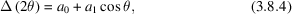which corrects for the zero-point error via the a0 term and, via the a1 cos θ term, for varying sample heights in reflection mode, or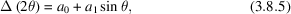which corrects for transparency errors, for example, andwhich provides transparency coupled with thick specimen error corrections, where a0 and a1 are constants that can be determined by shifting patterns to maximize their overlap as measured by ρ. It is difficult to obtain suitable expressions for the derivatives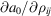and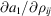for use in the optimization, so we use the downhill simplex method (Nelder & Mead, 1965), which does not require their calculation.

#### 3.8.2.5. Generation of the correlation and distance matrices

| top | pdf |

Using equation (3.8.3), a correlation matrix is generated in which a set of n patterns is matched with every other to give a symmetric (n × n) correlation matrix ρ with unit diagonal. The matrix ρ can be converted to a Euclidean distance matrix, d, of the same dimensions via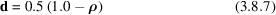or a distance-squared matrix,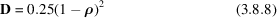for each entry i, j in d,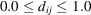. A correlation coefficient of 1.0 translates to a distance of 0.0, a coefficient of −1.0 to 1.0, and zero to 0.5. There are other methods of generating a distance matrix from ρ (see, for example, Gordon, 1981, 1999), but we have found this to be both simple and as effective as any other.

For other purposes a dissimilarity matrix s is also needed, whose elements are defined via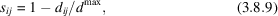where dmax is the maximum distance in matrix d. A dissimilarity matrix, δ, is also generated with elements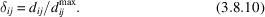In some cases it can be advantageous to use I1/2 in the distance-matrix generation; this can enhance the sensitivity of the clustering to weak peaks (Butler et al., 2019).

### References

Butler, B. M., Sila, A., Nyambura, K. D., Gilmore, C. J., Kourkoumelis, N. & Hillier, S. (2019). Pre-treatment of soil X-ray powder diffraction data for cluster analysis. Geoderma, 337, 413–424.Google Scholar
Donoho, D. L. & Johnstone, I. M. (1995). Adapting to unknown smoothness via wavelet shrinkage. J. Am. Stat. Assoc. 90, 1200–1224.Google Scholar
Gilmore, C. J., Barr, G. & Paisley, W. (2004). High-throughput powder diffraction. I. A new approach to qualitative and quantitative powder diffraction pattern analysis using full pattern profiles. J. Appl. Cryst. 37, 231–242.Google Scholar
Gordon, A. D. (1981). Classification, 1st ed., pp. 46–49. London: Chapman and Hall.Google Scholar
Gordon, A. D. (1999). Classification, 2nd ed. Boca Raton: Chapman and Hall/CRC.Google Scholar
Nelder, J. A. & Mead, R. (1965). A simplex method for function minimization. Comput. J. 7, 308–313.Google Scholar
Ogden, R. T. (1997). Essential Wavelets for Statistical Applications and Data Analysis, pp. 144–148. Boston: Birkhäuser.Google Scholar
Press, W. H., Teukolsky, S. A., Vetterling, W. T. & Flannery, B. P. (2007). Numerical Recipes. 3rd ed. Cambridge University Press..Google Scholar
Smrčok, Ĺ., Ďurík, M. & Jorík, V. (1999). Wavelet denoising of powder diffraction patterns. Powder Diffr. 14, 300–304.Google Scholar
Spearman, C. (1904). The proof and measurement of association between two things. Am. J. Psychol. 15, 72–101.Google Scholar